# Pipes and Cisterns Formulas Concepts and Questions for CAT Exam

Pipes and Cisterns Formulas Concepts and Questions for CAT Exam
4.8 (96%) 25 vote[s]

## Pipes and Cisterns basic concepts

We employ the same basic concepts of ‘Time and Work’ to solve questions based on pipes and cisterns. However, in some instances in addition to inlet pipes, the question also mentions outlet pipes.

A pipe connected to the cistern is called an inlet pipe or an outlet pipe, accordingly as it fills it or empties it respectively.

To make the principle of work and time to work here, we assume that the inlet pipes are doing positive work and the outlet pipes are doing negative work.

Let us take some varieties of questions to understand the method, tricks, and shortcuts to solve them.### Working with concepts on Pipes and Cisterns problems

Question 1: Two pipes A and B can fill a cistern in 20 and 30 minutes respectively, and a third pipe C can empty it in 40 minutes. How much time will it take to fill the cistern if all the three are opened at the same time?

Solution: LCM of 20, 30, and 40 = 120. Let us assume that the capacity of the cistern is 120 liters.
Therefore,

Rate of pipe A = 120/20 = 6 litres/min

Similarly, Rate of pipe B = 120/30 = 4 liters/min

And rate of pipe C = 120/40 = 3 liters/min

All three pipes are opened simultaneously, then in a min, the amount of water in the cistern in a min will be =6+4-3 =7 liters. As pipe C is an outlet pipe, we are taking its work to be negative.

Hence, the time taken to fill the cistern = 120/7 min.

So, from the above explanation its apparent that the problems from pipes and cisterns are an extension of work and time.

Now let us focus on some regular questions which generally appear and how we can apply pipes and cisterns formulas and tricks to solve the problems.

## Pipes and Cisterns Shortcut Tricks

### The time required by leak alone to empty the cistern

Important: If an inlet pipe takes X min to fill a cistern, but due to leak, it takes Y extra min to fill the cistern, then the amount of time in which the leak can empty the full cistern with the inlet pipe, not in operation will be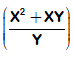min.

Question 2: If a cistern generally takes 20 min to be filled by a pipe, but due to leak, it takes 10 extra min to be filled, then the amount of time in which the leak can empty the full cistern is:

Normal Method:

Inlet pipe alone takes 20 min.

(Inlet pipe + Leak) take 20+10 = 30 min.

LCM of 20 and 30 = 60. Let the capacity of the cistern be 60 liters.

Rate per minute of inlet pipe = 60/20 = 3 liters/min ….. (1)

Rate per minute of inlet + leak = 60/30 = 2 liters/min ….(2)

solving (1) and (2) we get the rate per minute of leak = -1 liters/min

Therefore, when the inlet is non-operational, the leak will take 60/1 = 60 minutes or one hour to empty the full cistern.

Shortcut Trick and Formula:

On comparing, we have X=20 and Y=10. Therefore, the leak will take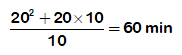### Finding the capacity of the cistern

Important:  A cistern has a leak which can empty it in X hours. A pipe which admits Y liters of water per hour into the cistern is turned on, and now the cistern is emptied in Z hours. Then the capacity of the cistern is liters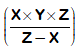.

Question 3: A cistern has a leak which can empty it in 4 hours. A pipe which admits 20 liters of water per hour into the cistern is turned on, and now the cistern is emptied in 6 hours. What is the capacity of the cistern?

Normal Method:

Let the capacity of the cistern be x liters.

Therefore, the rate per hour of the leak when the inlet pipe is not operating = x/4.

Now, when the inlet pipe is operational, the leak takes 6 hours to empty the cistern.
Therefore, the rate per hour of the leak when the inlet pipe is not operating= x/6.

As a result, we have the following relation:

x/4-20=x/6.
On solving the above equation, we get x = 240 liters.

Shortcut Trick and Formula:

By comparing with the shortcut formula, X=4, Y=20, and Z=6. Therefore, the capacity of the cistern =Or the capacity of the cistern = 240 liters.

### Pipes opened for different durations.

Important: If two pipes A and B are opened for different time periods, then to solve the question we use:

A’s amount of work +B’s amount of work=1.

Or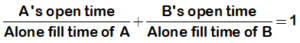Question 4: Two pipes A and B can fill a cistern in 12 and 16 min respectively. Both pipes are opened together but 4 min before the cistern is full, one pipe A is closed. Find the time required to fill the cistern.

Shortcut Trick and Formula:

Let it takes x min by the pipes A and B to fill the cistern.

Therefore, the duration for which pipe was operating = (x-4) min. And pipe B was operating for x min.

Now, substituting in the formula given above, we get: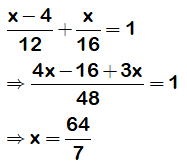Hence, It will take 64/7 min to fill the cistern.

### The rate of flow of the pipe is proportional to the diameter.

Question 5: There are three inlet pipes whose diameters are 1 cm, 2 cm, and 4 cm respectively. The rate of flow of water is directly proportional to the square of the diameter. The pipe with the largest diameter takes 16 min to fill a cistern. How much time it will take for all three pipes, working together, to fill the cistern.

Explanation:

Let the three pipes be A, B, and C respectively.

Therefore,
For pipe A, the rate of flow per min = k(1)2 = k

Similarly, the rate of flow per min of pipe B = k(2)2 =4k
And, the rate of flow per min of pipe C = k(4)2 =16k, where k is a proportionality constant.

It is given that the pipe C can alone fill the cistern in 16 min.

Hence, the capacity of cistern = the rate of flow of pipe C×time taken by the pipe C=16k×16=256k liters.

Now, when all three pipes operate together, the combined rate of flow per min = k+4k+16k=21k.

Therefore, the time required to fill the cistern = 256k/21k=256/21 minutes.

### Additional Questions on pipes and cisterns for CAT Exam

Question 6: A cistern can be filled by two taps A and B in 25 minutes and 30 minutes respectively and can be emptied by a third in 15 minutes. If all the taps are turned on at the same moment, what part of the system will remain unfilled at the end of 100 minutes?

Explanation:

LCM of 25 30 and 15 equals 150.

Assume that the capacity of the cistern =150 litres.

The rate of tap A= 150/25=6 litres per minute.

Similarly, the rate of tap B and C 5 and 10 litres per minute respectively.

The rate at which the cistern is filled per min = 6+5-10 =1 litre per min.

So, the time required to fill the cistern completely = 150/1 equals 150 minutes.

Therefore, the time taken to fill the cistern with 100 Litres = 100/1 = 100 minutes.

As a result, 150-100 = 50 litres is still remaining to fill the cistern completely.

Or you can say the portion of the cistern which is unfilled = 50/150 = 1/3.

Question 7:  A swimming pool is fitted with three pipes. The first two pipes working simultaneously, fill the pool at the same time as the third pipe alone. The second pipe alone fills the pool in 5 hours faster than the first pipe and 4 hours slower than the third pipe. In how much time will the second and the third pipes together fill the pool?

Explanation:

Let V be the volume of the pool and x be the time in which the second pipe can fill the pool. The first pipe takes x + 5 hours, the third pipe takes x-4 hours.

Then as per the condition, we have the following relation:

V/(x+5)+V/x=V/(x-4)

On solving for the above equation, we get x = 10.

Therefore, 1/10 + 1/6 = 16/60 of the pool will be filled second and third pipe together in one hour.

To fill the pool, they take 60/16 =3.75 hours.

Question 8:  There is a leak in the bottom of a cistern. When the cistern had no leak, it was filled in 2.5 hours. It now takes half an hour longer. If the cistern is full of water, then how long would it take to empty itself if water starts leaking out at the double the usual rate once the cistern is half empty?

Explanation:

The inlet pipe can fill the cistern in 2.5 hours when the leak is not operating.

When the leak is operating along with the inlet pipe, now it takes cheers to fill the cistern.

LCM of 2.5 and 3= 15

Let the capacity of the cistern = 15 litres.

The rate of inlet pipe=15/2.5 = 6 litres per hour.

The rate of the inlet pipe and the leak together=15/3=5 litres per hour.

That means the rate of the leak = 6-5 equals 1 litre per hour.

So the time required to empty the cistern to half when only the leak is operating = 7.5/1=7.5 hours.

Now,  the new rate of the leak = 2 litres per hour.

So that time required fully empty the cistern from half filled = 7.5/2 = 3.75 hours.

Therefore, the total time required = 7.5 + 3.75 =11.25 hours =11 hours 15 minutes.

### Final takeaways:

From the above examples, we can conclude that the necessary concepts used to solve problems from pipes and cisterns remain the same as the concepts of work and time. Although depending upon the variety, we can use the available shortcuts or tricks to manage the questions on pipes and cisterns in quick time.